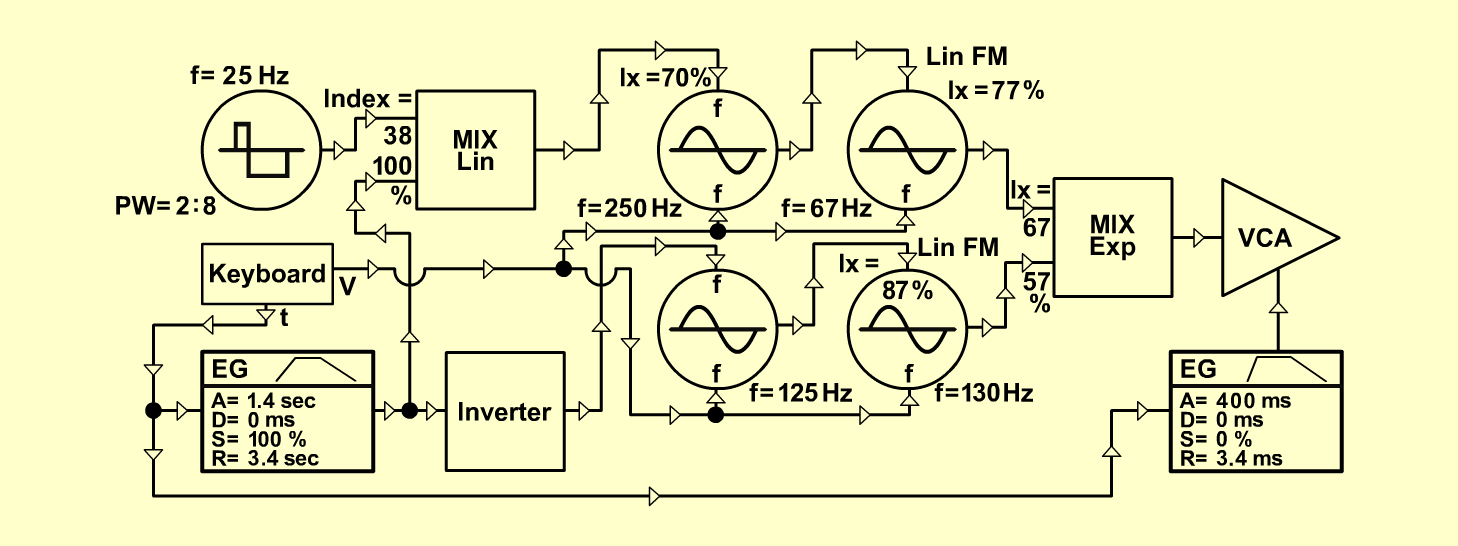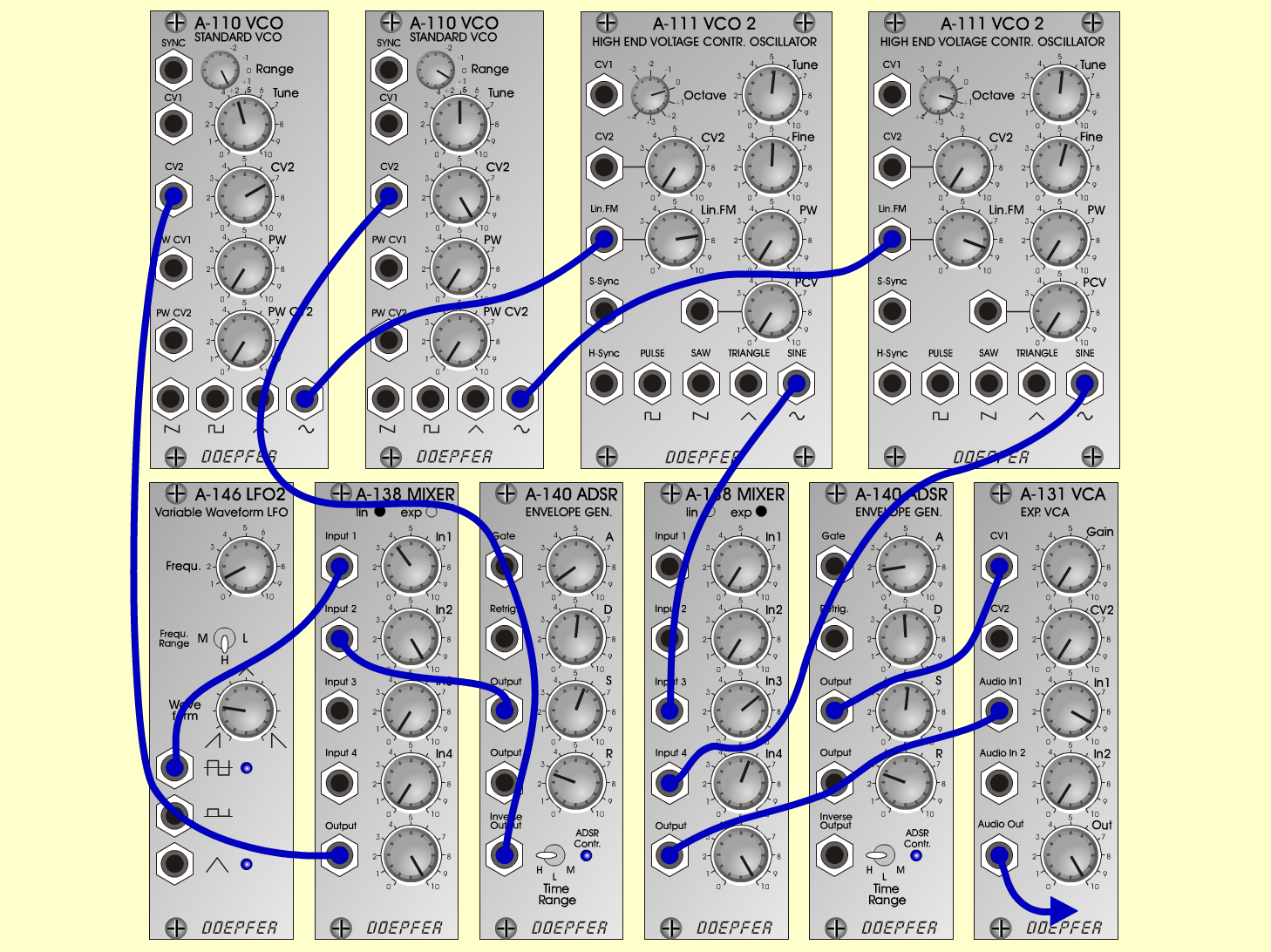FMscapeFXD o e p f e r A - 100 Connections: Settings: A-110/1 (Sine)  <=>  A-111/1 (LinFM) A-110/2 (Sine)  <=>  A-111/2 (LinFM) A-111/1 (Sine)  <=>  A-138exp (Input 3) A-111/2 (Sine)  <=>  A-138exp (Input 4) A-138exp (Output)  <=>  A-131 (Audio In) A-131 (Audio Out)  <=> Amplifier A-140/1 (Output)  <=>  A-131 (CV 1) A-140/2 (Output)  <=>  A-138lin (Input 2) A-140/2 (Inv. Output)  <=>  A-110/2 (VC 2) A-146 (Pulse/AC)  <=>  A-138lin (Input 1) A-138lin (Output)  <=>  A-110/1 (CV 2) KB (Gate)  <=>  A-185 (Gate In) KB (CV)  <=>  A-185 (CV In) A-185 (Gate Out / Bus)  <=>  A-140/1+2 (Gate) A-185 (CV Out / Bus)  <=>  A-110/1+2 (CV) A-185 (CV Out / Bus)  <=>  A-111/1+2 (CV) A-110/1 (Range = 2, Tune = 4.5, CV 2 = 7) A-110/2 (Range = 1, Tune = 5, CV 2 = 10) A-111/1 (Oct = 0, Tune = 5.2, Fine = 5.1, Lin FM = 7.7) A-111/2 (Oct = 1, Tune = 5.2, Fine = 5.5, Lin FM = 8.7) A-138exp (In 3 = 6.7, In 4 = 5.7, Out = 10) A-131 (Gain = 0, Audio In 1 = 9,  Audio Out = 10) A-140/1 (A = 0.8, D = 5.2, S = 5.7, R = 2.7, Range = H) A-140/2 (A = 1.7, D = 4.9, S = 5.2, R = 2.7, Range = H) A-138lin (In 1 = 3.8, In 2 = 10, Out = 10) A-146 (Freq = 1.1, Wave Form = 2.3, Range = H) Bakis Sirros, January 2002  group: PARALLEL WORLDSSound sample FMscape